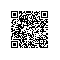# 经典算法题每日演练——第十八题 外排序

说到排序，大家第一反应基本上是内排序，是的，算法嘛，玩的就是内存，然而内存是有限制的，总有装不下的那一天，此时就可以来玩玩

1.概序

我们知道算法中有一种叫做分治思想，一个大问题我们可以采取分而治之，各个突破，当子问题解决了，大问题也就KO了，还有一点我们知道

TopN条记录，然后我们从M路队列中各取一个数字进入中转站队列，并将该数字打上队列编号标记，当从中转站出来的最小数字就是我们最后要排2.构建

<1> 生成数据

这个基本没什么好说的，采用随机数生成n条记录。

#region 随机生成数据
/// <summary>
/// 随机生成数据
///<param name="max">执行生成的数据上线</param>
/// </summary>
public static void CreateData(int max)
{
var sw = new StreamWriter(Environment.CurrentDirectory + "//demo.txt");

for (int i = 0; i < max; i++)
{
var rand = new Random((int)DateTime.Now.Ticks).Next(0, int.MaxValue >> 3);

sw.WriteLine(rand);
}
sw.Close();
}
#endregion

<2> 切分数据
根据实际情况我们来决定到底要分成多少个小文件，并且小文件的数据必须是有序的，小文件的个数也对应这内存中有多少个优先队列。

#region 将数据进行分份
/// <summary>
/// 将数据进行分份
/// <param name="size">每页要显示的条数</param>
/// </summary>
public static int Split(int size)
{
//文件总记录数
int totalCount = 0;

//每一份文件存放 size 条 记录
List<int> small = new List<int>();

var sr = new StreamReader((Environment.CurrentDirectory + "//demo.txt"));

var pageSize = size;

int pageCount = 0;

int pageIndex = 0;

while (true)
{

if (!string.IsNullOrEmpty(line))
{
totalCount++;

//加入小集合中

//说明已经到达指定的 size 条数了
if (totalCount % pageSize == 0)
{
pageIndex = totalCount / pageSize;

small = small.OrderBy(i => i).Select(i => i).ToList();

File.WriteAllLines(Environment.CurrentDirectory + "//" + pageIndex + ".txt", small.Select(i => i.ToString()));

small.Clear();
}
}
else
{
//说明已经读完了，将剩余的small记录写入到文件中
pageCount = (int)Math.Ceiling((double)totalCount / pageSize);

small = small.OrderBy(i => i).Select(i => i).ToList();

File.WriteAllLines(Environment.CurrentDirectory + "//" + pageCount + ".txt", small.Select(i => i.ToString()));

break;
}
}

return pageCount;
}
#endregion

<3> 加入队列

我们知道内存队列存放的只是小文件的topN条记录，当内存队列为空时，我们需要再次从小文件中读取下一批的TopN条数据，然后放入中转站

#region 将数据加入指定编号队列
/// <summary>
/// 将数据加入指定编号队列
/// </summary>
/// <param name="i">队列编号</param>
/// <param name="skip">文件中跳过的条数</param>
/// <param name="list"></param>
/// <param name="top">需要每次读取的条数</param>
public static void AddQueue(int i, List<PriorityQueue<int?>> list, ref int[] skip, int top = 100)
{
var result = File.ReadAllLines((Environment.CurrentDirectory + "//" + (i + 1) + ".txt"))
.Skip(skip[i]).Take(top).Select(j => Convert.ToInt32(j));

//加入到集合中
foreach (var item in result)
list[i].Eequeue(null, item);

//将个数累计到skip中，表示下次要跳过的记录数
skip[i] += result.Count();
}
#endregion

<4> 测试

最后我们来测试一下:

数据量：short.MaxValue。

内存存放量：1200。

public static void Main()
{
//生成2^15数据
CreateData(short.MaxValue);

//每个文件存放1000条
var pageSize = 1000;

//达到batchCount就刷新记录
var batchCount = 0;

//判断需要开启的队列
var pageCount = Split(pageSize);

//内存限制：1500条
List<PriorityQueue<int?>> list = new List<PriorityQueue<int?>>();

//定义一个队列中转器
PriorityQueue<int?> queueControl = new PriorityQueue<int?>();

//定义每个队列完成状态
bool[] complete = new bool[pageCount];

//队列读取文件时应该跳过的记录数
int[] skip = new int[pageCount];

//是否所有都完成了
int allcomplete = 0;

//定义 10 个队列
for (int i = 0; i < pageCount; i++)
{

//i：   记录当前的队列编码
//list: 队列数据
//skip：跳过的条数
}

//初始化操作，从每个队列中取出一条记录，并且在入队的过程中
//记录该数据所属的 “队列编号”
for (int i = 0; i < list.Count; i++)
{
var temp = list[i].Dequeue();

//i:队列编码,level:要排序的数据
queueControl.Eequeue(i, temp.level);
}

//默认500条写入一次文件
List<int> batch = new List<int>();

//记录下次应该从哪一个队列中提取数据
int nextIndex = 0;

while (queueControl.Count() > 0)
{
//从中转器中提取数据
var single = queueControl.Dequeue();

//记录下一个队列总应该出队的数据
nextIndex = single.t.Value;

var nextData = list[nextIndex].Dequeue();

//如果改对内弹出为null，则说明该队列已经，需要从nextIndex文件中读取数据
if (nextData == null)
{
//如果该队列没有全部读取完毕
if (!complete[nextIndex])
{

//如果从文件中读取还是没有，则说明改文件中已经没有数据了
if (list[nextIndex].Count() == 0)
{
complete[nextIndex] = true;
allcomplete++;
}
else
{
nextData = list[nextIndex].Dequeue();
}
}
}

//如果弹出的数不为空，则将该数入中转站
if (nextData != null)
{
//将要出队的数据 转入 中转站
queueControl.Eequeue(nextIndex, nextData.level);
}

//如果batch=500，或者所有的文件都已经读取完毕，此时我们要批量刷入数据
if (batch.Count == batchCount || allcomplete == pageCount)
{
var sw = new StreamWriter(Environment.CurrentDirectory + "//result.txt", true);

foreach (var item in batch)
{
sw.WriteLine(item);
}

sw.Close();

batch.Clear();
}
}

Console.WriteLine("恭喜，外排序完毕！");
}使用钉钉扫一扫加入圈子
+ 订阅